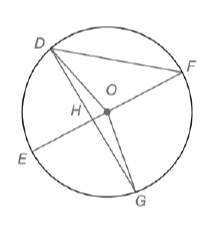Chapter 6.1, Problem 12EElementary Geometry For College St...

7th Edition
Alexander + 2 others
ISBN: 9781337614085

Solutions

Chapter
SectionElementary Geometry For College St...

7th Edition
Alexander + 2 others
ISBN: 9781337614085
Textbook Problem

Given: m ∠ D O E = 76 ∘ and m ∠ E O G = 82 ∘ in ⊙ O E F ¯  is a diameterFind: a) m D E ⌢ b) m D F ⌢ c) m ∠ F d) m ∠ D G E e) m ∠ E H G f) Whether m ∠ E H G = 1 2 ( m E G ⌢ + m D F ⌢ )

To determine

(a)

To calculate:

The mDE by using the provided figure.

Explanation

Given:

mDOE=76o and mEOG=82o in Q. EF¯ is a diameter.

Postulate used:

Central angle postulate:

In a circle, the degree of a central angle is equal to the degree measure of its intercepted arc.

Calculation:

Since, mDOE=76o

To determine

(b)

To calculate:

The mDF by using the provided figure.

To determine

(c)

To calculate: The F by using the provided figure.

To determine

(d)

To calculate:

The mDGE by using the provided figure.

To determine

(e)

To calculate:

The mEHG by using the provided figure.

To determine

(f)

To calculate:

The mEHG=12(mEG+mDF) by using the provided figure.

Still sussing out bartleby?

Check out a sample textbook solution.

See a sample solution

The Solution to Your Study Problems

Bartleby provides explanations to thousands of textbook problems written by our experts, many with advanced degrees!

Get Started

Find more solutions based on key concepts